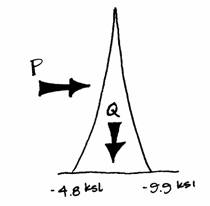Internal Stresses

The values of compressive and tensile stress can be found from the formula:where the force, N, is the total axial force found from both vertical and horizontal loads.  Again, negative numbers are compressive and positive tensile.  In the idealization of the Tower’s geometry, the area of each of the two simplified columns is 1600 square inches.  The compression forces found at the bases of the Tower’s columns are N = -7,630 kips on the windward side, and N = -15,780 kips on the leeward side.  The resulting stresses are:on the windward side and:on the leeward side.Because the wind may act on the Tower from any direction, each of the supports must be designed to take the maximum stress of -9.9 ksi.  This is also a rough estimate of the maximum stress on each of the actual Tower’s four columns, because the idealization has combined four columns into two twice as large.

Structural Studies Home